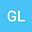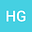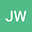Long time behavior of stochastic evolution equations
•••Hongjun Gao
Nanjing Normal University
Author ProfileJinlong Wei
Zhongnan University of Economics and Law
Author Profile## Abstract

In this paper, we consider the long time behavior of stochastic evolution equations. The exponential, polynomial and logarithmic decay for stochastic equations are considered. Sufficient conditions are given to obtain these exponents. All the results show the noise (time diffusion) will prevent the solutions to decay in $p$-th moment, which coincides with the fact that the noise is a diffusion process but it will be different in the sense of almost surely, and the partial diffusion operator (spatial diffusion) will accelerate the decay of solutions.